Students can download Maths Chapter 4 Symmetry Ex 4.1 Questions and Answers, Notes, Samacheer Kalvi 6th Maths Guide Pdf helps you to revise the complete Tamilnadu State Board New Syllabus, helps students complete homework assignments and to score high marks in board exams.

## Tamilnadu Samacheer Kalvi 6th Maths Solutions Term 3 Chapter 4 Symmetry Ex 4.1

Question 1.
Fill in the blanks
(i) The reflected image of the letter ‘q’ is …….
(ii) A rhombus has ………… lines of symmetry.
(iii) The order of rotational symmetry of the letter ‘Z’ is ……….
(iv) A figure is said to have rotational symmetry, if the order of rotation is atleast ……….
(v) ……… symmetry occurs when an object slides to new position.
Solution:
(i) P
(ii) two
(iii) 2
(iv) two
(v) TranslationQuestion 2.
Say True or False
(i) A rectangle has four lines of symmetry.
(ii) A shape has reflection symmetry if it has a line of symmetry.
(iii) The reflection of the name RANI is INAЯ.
(iv) Order of rotation of a circle is infinite.
(v) The number 191 has rotational symmetry.
Solution:
(i) False
(ii) True
(iii) False
(iv) True
(v) False

Question 3.
Match the following shapes with their number of lines of symmetry.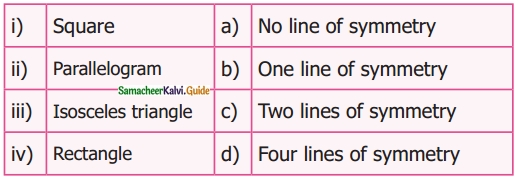Solution:
(i) d
(ii) a
(iii) b
(iv) cQuestion 4.
Draw the lines of symmetry of the following.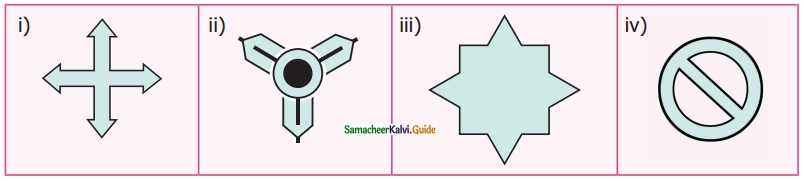Solution: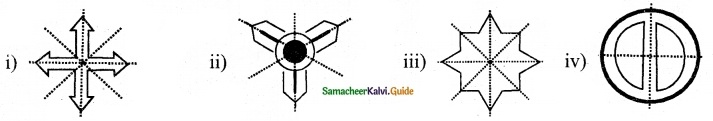Question 5.
Using the given horizontal line/ vertical line as a line of symmetry, complete each alphabet to discover the hidden word.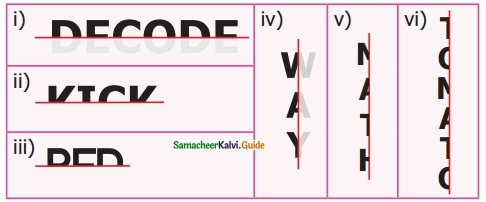Solution:
(i) DECODE
(ii) KICK
(iii) BED
(iv) WAY
(v) MATY
(vi) TOMATOQuestion 6.
Draw a line of symmetry of the given figures such that one hole coincides with the other hole(s) to make pairs.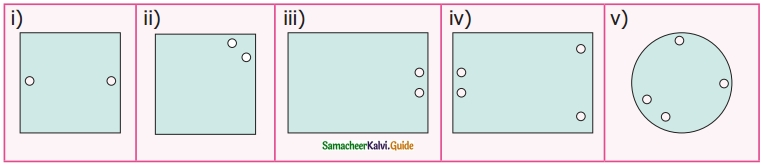Solution: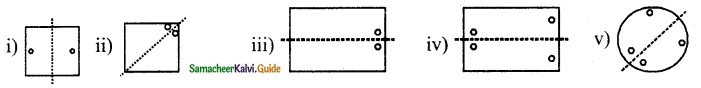Question 7.
Complete the other half of the following figures such that the dotted line is the line of symmetry.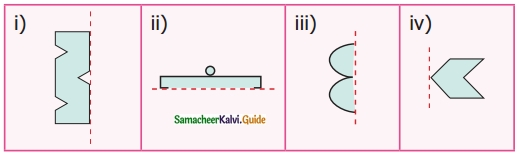Solution: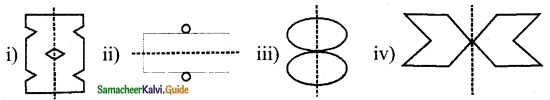Question 8.
Find the order of rotation for each of the following.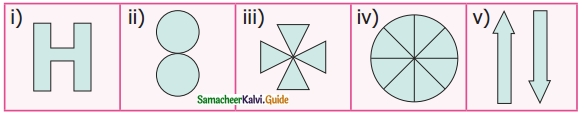Solution:
(i) 2
(ii) 2
(iii) 4
(iv) 8
(v) 2

Question 9.
A standard die has six faces which are shown below. Find the order of rotational symmetry of each face of a die?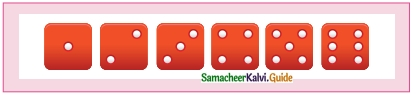Solution:
(i) 4
(ii) 2
(iii) 2
(iv) 4
(v) 4
(vi) 2Question 10.
What pattern is translated in the given border kolams?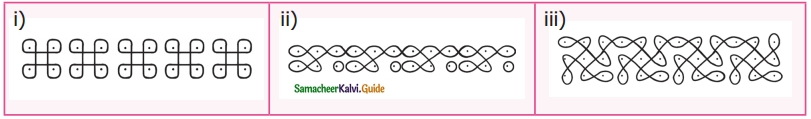Solution: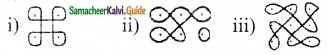Objective Type Questions

Question 11.
Which of the following letter does not have a line of symmetry?
(a) A
(b) P
(c) T
(d) U
Hint: A, T, U have one line of symmetry
Solution:
(b) PQuestion 12.
Which of the following is a symmetrical figure?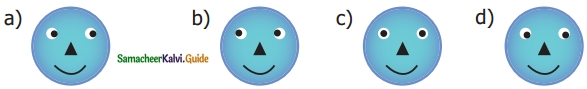Solution:
(c)

Question 13.
Which word has a vertical line of symmetry?
(b) NUN
(c) MAM
(d) EVE
Hint: D, N, E have no vertical line of symmetry
Solution:
D, N, E have no vertical line of symmetry

Question 14.
The order of rotational symmetry of 818 is ………
(a) 1
(b) 2
(c) 3
(d) 4
Solution:
(b) 2Question 15.
The order of rotational symmetry ★ is ___
(a) 5
(b) 6
(c) 7
(d) 8
Solution:
(a) 5